# Part 4: Angle RelationshipsAngle relationships is an essential topic because it forms the foundation of many different Maths topics that you will come across in the future. In this guide, we will give you the best tips to work with angle relationships.

## Syllabus Outcomes

We will be looking at NSW Syllabus Outcomes:

 NSW Stage 4 Syllabus Outline Syllabus Explanation Demonstrate that the angle sum of a triangle is 180o and use this to find the angle sum of a quadrilateral (ACMMG166) This means that you will know how to identify and figure out angle sizes in supplementary angles. Establish properties of quadrilaterals using congruent triangles and angle properties, and solve related numerical problems using reasoning (ACMMG202) This means that you know how to use different angles on a point and angles associated with parallel lines. Identify, name and measure alternate angle pairs, corresponding angle pairs and co-interior angle pairs for two lines cut by a transversal (ACMMG163) This means that you can work out sizes on different angles by looking at alternate, corresponding and co-interior angle pairs. Solve simple numerical problems using reasoning (ACMMG164) This means that you are able to questions like: “Find the value of $$\alpha°$$ in the diagram”

## Why do students struggle with Angle Relationships?

Understanding and learning angle relationships may be easy… But the majority of students face difficulty when applying angle relationships.

They also struggle with presenting answers in a coherent way that’s not obtuse. This means that markers will struggle to follow the reasoning provided.

These are the main difficulties faced by students in this topic:

• Providing a coherent set of reasons that a marker would understand
• Writing out the complete reason for a certain angle relationship.

This guide will help you overcome these difficulties, as well as covering the following content:

• Naming lines and angles
• Complementary angles, supplementary angles and angles about a point
• Vertically opposite angles
• Angle relationships on parallel lines

## Assumed Knowledge

To do well in Angle Relationships, you need to know about:

### Naming Lines and Angles

Students should be familiar with how to name lines as well as angles.

Lines should be named as a group pair of letters.

For example, the diagram below shows the lines $$AB$$.To name angles, identify the vertex and the two letters at the end of each ray.

In the case below,

• $$B$$ is the vertex
• $$A$$ and $$B$$ are the ends of each ray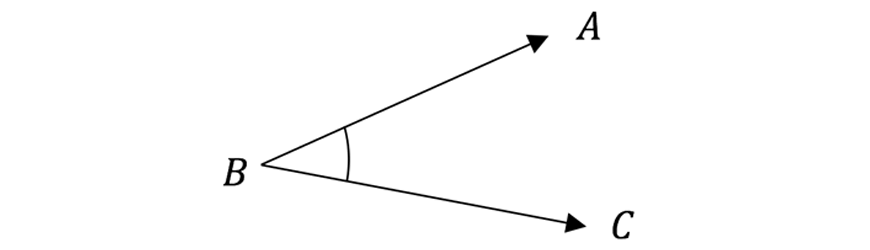To name the angle above, the middle letter should represent its vertex.

Thus the angle can be named as such:

\begin{align*}
\angle ABC \ or \ \angle CBA \ or \ \angle B
\end{align*}

## Angles from a point

### Complementary Angles

Two angles that add up to $$90º$$ are called complementary angles.

• The angles, $$\alpha$$ and $$\beta$$ are complementary angles, where $$\alpha + \beta = 90º$$### Supplementary Angles

Similarly, two angles that add up to $$180º$$ are called supplementary angles.

• The angles $$\alpha$$ and $$\beta$$ are supplementary angles, where $$\alpha + \beta = 180º$$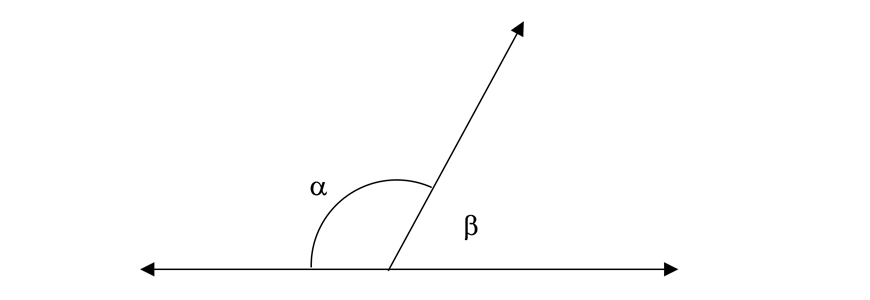### Angles at a Point (Angles in a revolution)

Angles at a point form a revolution add up to $$360°$$.

In the diagram below:

• $$\alpha + \beta + \gamma + \delta = 360º$$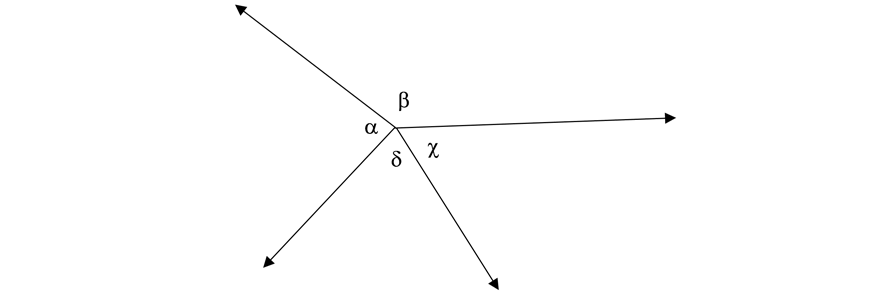Example

1. Find the size of $$x$$.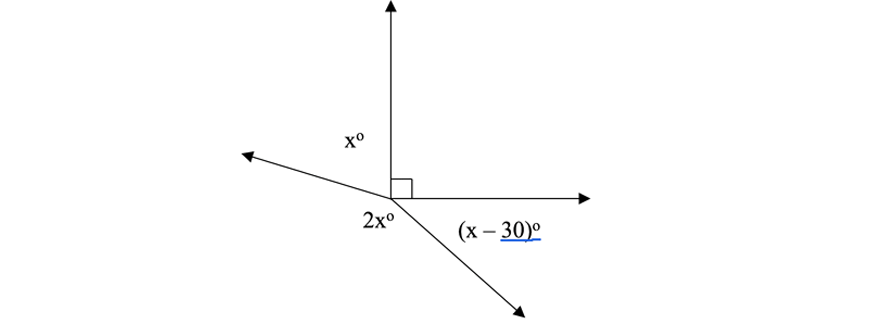Solution

To determine the size of $$x$$, we need to utilise the fact that angles at a point add to  $$360°$$.

As a result,

\begin{align*}
x°+ 2x° + (x-30)° + 90° &= 360° \ (\text{Angles of a revolution add to} \ 360°) \\
4x° – 30° + 90° &= 360° \\
4x° &= 300° \\
∴ x° &= 75º
\end{align*}

### Vertically Opposite Angles

When two straight lines intersect, the two pairs of opposite angles formed are equal.

These are known as vertically opposite angles.

In the diagram below:

• $$AD$$ and $$BC$$ intersect at the point $$X$$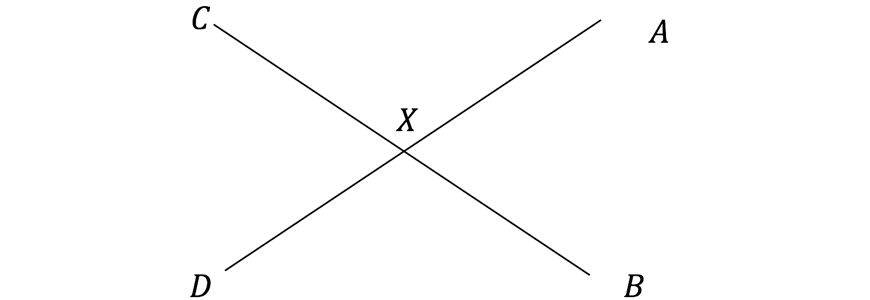• $$\angle CXA = \ \angle DXB \ (\text{Vertically opposite angles are equal})$$
• $$\angle CXD = \ \angle AXB \ (\text{Vertically opposite angles are equal})$$

## Angles Associated with Parallel Lines

### Parallel Lines

Parallel lines are lines in a plane that do not intersect or touch each other at any point.

Notation: Parallel lines are marked with arrowheads pointing in the same direction.

In the diagram:

• $$AB$$ and $$CD$$ and parallel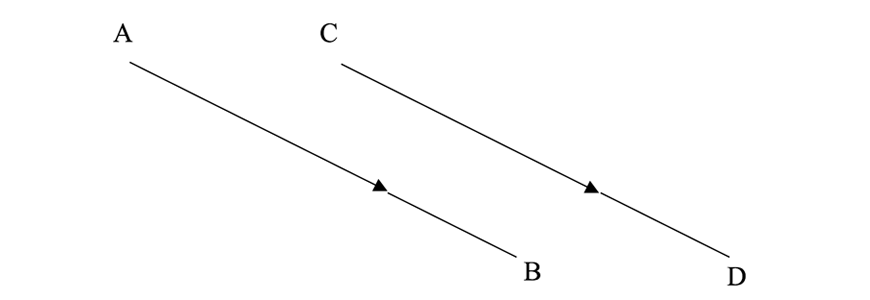To represent that $$AB$$ and $$CD$$ are parallel, we use the parallel symbol and notate as $$AB \parallel CD$$

A transversal is a line that crosses two or more lines.

In the diagram, the transversal $$EF$$ cuts the lines $$AB$$ and $$CD$$.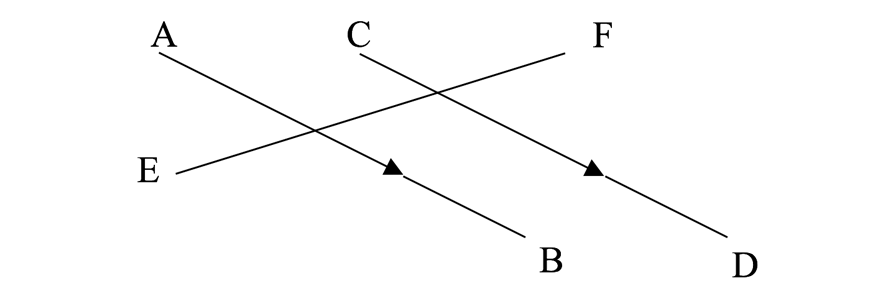Transversals which cross parallel lines, give rise to three fundamental parallel angle relationships (Alternate, Corresponding and Co-interior angles).

### Alternate Angles

Alternate angles are angles on opposite sides of the transversal and between the parallel lines.

Alternate angles on parallel lines are equal.

In the diagram below,

• $$\alpha$$ and $$\beta$$ are equal in size.

An example of written reasoning could be,

$$\angle AXY = \angle XYD \ (\text{Alternate angles on parallel lines} \ AB \ \text{and} \ CD \ \text{are equal})$$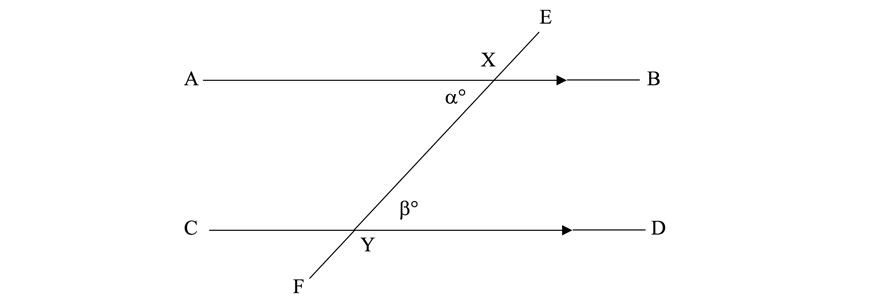• Alternate angles can be identified by a $$“Z”$$ orientation as shown in the bold lines in the diagram below.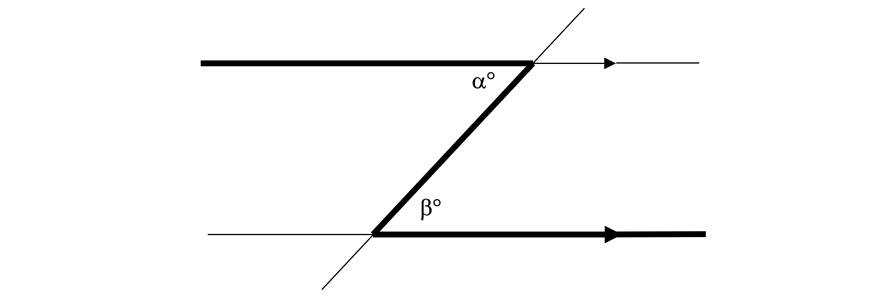## Corresponding Angles

Corresponding angles are angles on same sides of the transversal. One angle is between the parallel lines while the other is outside. Corresponding angles on parallel lines are equal.

In the diagram below:

• $$\alpha$$ and $$\beta$$ are equal in size.

An example of written reasoning could be,

$$\angle AXY = \angle CYF \ (\text{Corresponding angles on parallel lines} \ AB \ \text{and} \ CD \ \text{are equal})$$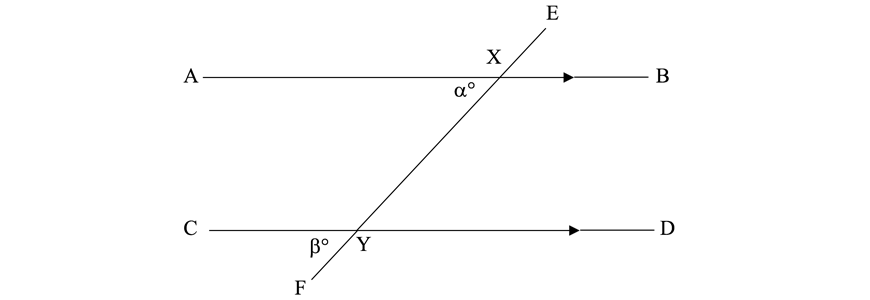• Corresponding angles can be identified by a $$“F”$$ orientation as shown in the bold lines in the diagram below.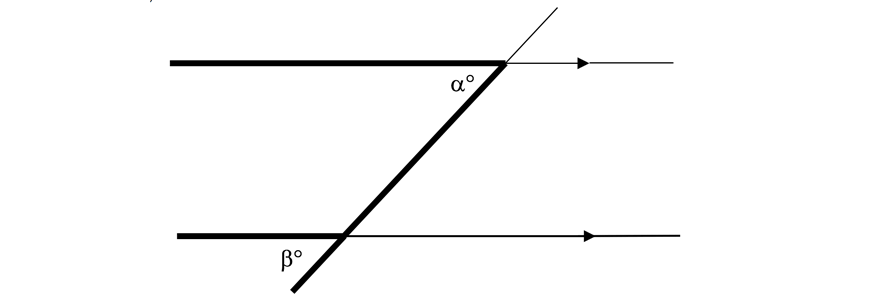## Co-Interior Angles

Co-interior angles are angles on same sides of the transversal between the parallel lines.

Co-interior angles are supplementary (add up to $$180°$$)

In the diagram below:

• $$\alpha$$ and $$\beta$$ are supplementary angles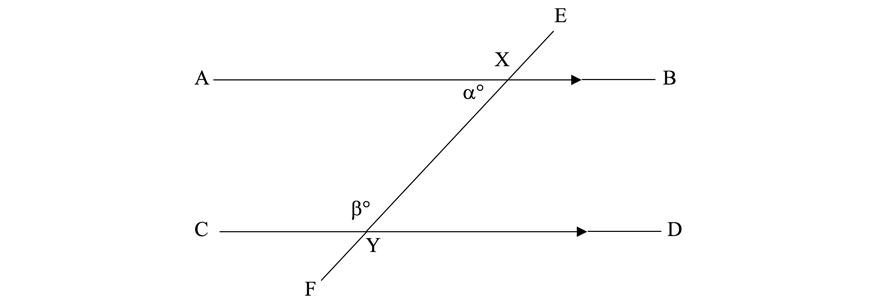An example of written reasoning could be:

• $$\angle AXY + \angle CYX = 180º \ (\text{Co-interior angles on parallel lines} \ AB \ \text{and} \ CD \ \text{are supplementary})$$

Co-interior angles can be identified by a “C” orientation as shown in the bold lines in the diagram below.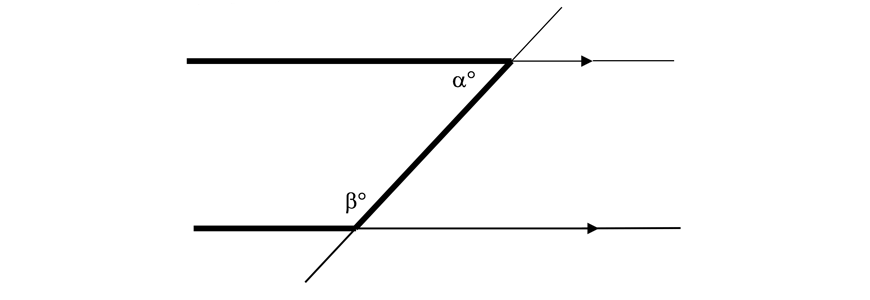Example

1. Find the value of $$\beta$$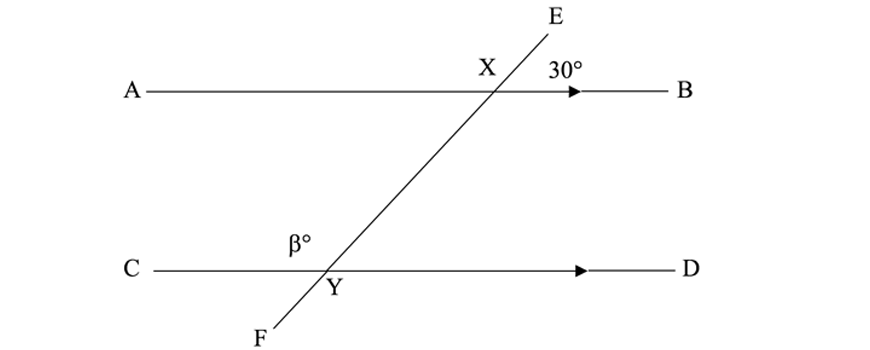Solution

\begin{align*}
\angle EXB &= \angle AXY = 30° \ (\text{Vertically opposite angles are equal}) \\
\beta° &= 180° – \angle AXY \ (\text{Co-interior angles on parallel lines are supplementary})\\
\beta° &= 180° – 30° \\
\beta° &= 150°
\end{align*}

## Proving Lines are Parallel

Conversely, we can use these angle relationships to prove that two lines are parallel.

This occurs when one or more of the following relationships hold:

• Alternate angles are equal
• Corresponding angles are equal
• Co-interior angles are supplementary

Example

1. Prove that $$AF$$ and $$CE$$ are parallel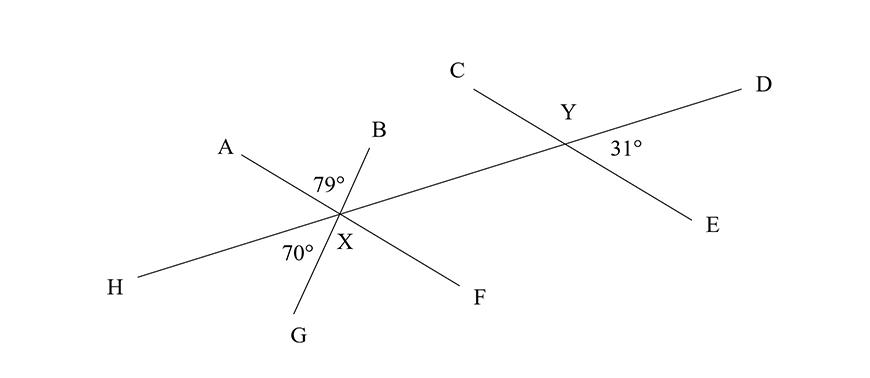Solution

\begin{align*}
<AXB &= \angle GXF = 79° \ \ (\text{Vertically opposite angles are equal}) \\
<YXF &= 180° – <HXG \ – \angle GXF \ \ (\text{Angle sum of straight lines}) \\
<YXF &= 180° – 70° – 79° \\
<YXF &= 31° \\
<YXF &= \angle DYE = 31°\\
∴ AF \ &and \ CE \ are \ parallel \ \ (\text{Corresponding angles} \ \angle YXF \ \text{and} \ \angle DYE \ \text{are equal})
\end{align*}

## Summary

1. To determine an angle given a diagram, first roughly work out the angle you’re trying to figure out.
2. Then, using the sample reasoning mentioned in the above content section, provide coherent reasoning to clearly list out steps required to reach the answer.

The following is a summary of the angle relationships covered in the post:

 Angle relationship Description Diagram Explanation Complementary angles Angles add to $$90°$$$$\alpha + \beta = 90°$$ Supplementary angles Angles add to $$180°$$$$\alpha + \beta = 180°$$ Angles at a point Angles add to $$360°$$$$\alpha + \beta + \gamma + \delta = 360°$$ Vertically opposite angles Angles are equal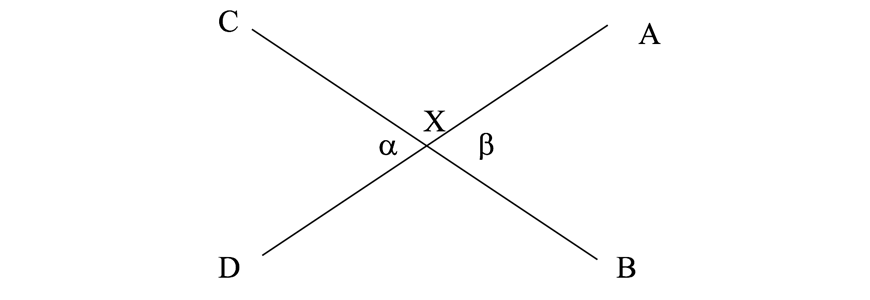$$\alpha = \beta$$ Alternate angles Angles are equal$$\alpha = \beta$$ Corresponding angles Angles are equal$$\alpha = \beta$$ Co-interior angles Angles are supplementary$$\alpha + \beta = 180°$$

## Checkpoint questions

1. Given that $$\alpha°$$ and $$\beta°$$ are complementary, what is value of $$\beta°$$ if  $$\alpha° = 54°$$

2. Find the value of $$\alpha°$$ and $$\beta°$$ in the following diagram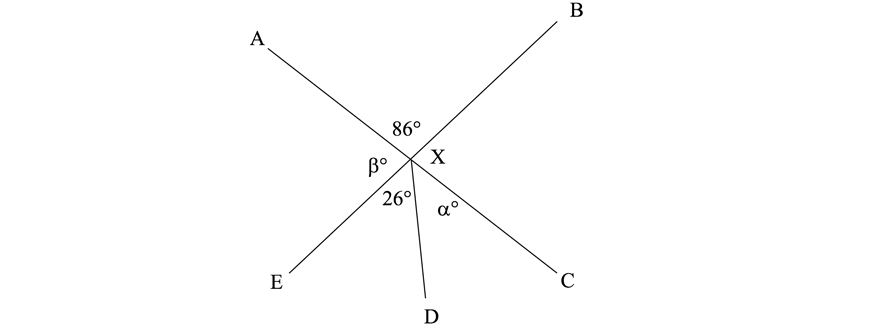3. Prove that $$BC$$ and $$DE$$ are parallel.

4. Find the size of $$\alpha°$$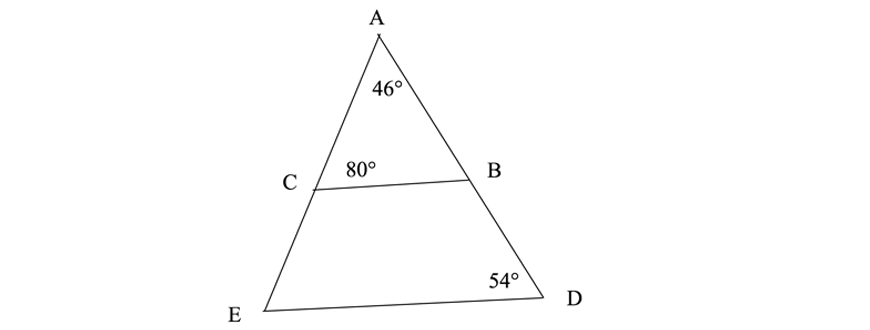5. Find the size of $$\alpha°$$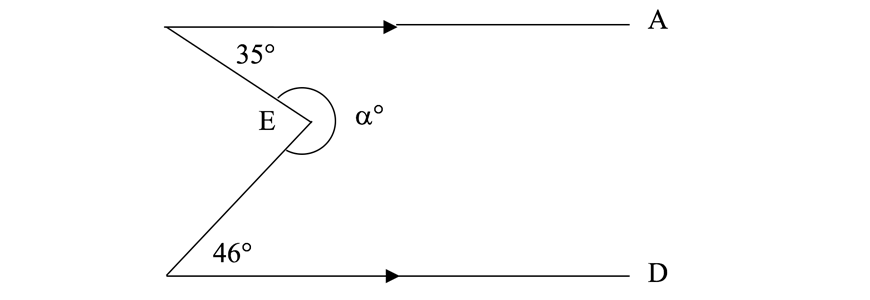## Solutions

1.

\begin{align*}
\beta° = 36°
\end{align*}

2.

\begin{align*}
\beta° &= 180° – \angle AXB \ (\text{Angle sum of straight line}) \\
\beta° &= 94° \\
\angle EXC &= \angle AXB = 86° \ (\text{Vertically opposite angles are equal}) \\
\alpha° + 26° &= 86° \\
\alpha° &= 60°
\end{align*}

3.

\begin{align*}
\angle{CBD} &= \angle{BAC} + \angle{ACB} \ (\text{Exterior angle sum of triangle}) \\
\angle{CBD} &= 126° \\
\angle{CBD} &+ \angle{BDE} \\
&= 126° + 54° \\
&= 180° \\
\end{align*}

\begin{align*}
∴ BC \ \text{and} \ DE \ \text{are parallel Co-interior angles , angles} \angle{CBD} \ \text{and} \ \angle{BDE} \text{are supplementary on parallel lines})
\end{align*}

4.

\begin{align*}
\angle CDA &= \angle DEB = 125° \ (\text{Corresponding angles on parallel lines} \ AK \ \text{and} \ BL \ \text{are equal}) \\
\angle DEB &= \angle HIE = 125° \ (\text{Corresponding angles on parallel lines} \ CF \ \text{and} \ GJ \ \text{are equal}) \\
\alpha° & = \angle HIE = 125° \ (\text{Vertically opposite angles are equal})
\end{align*}

5. Construct $$EF$$ so that it is parallel to $$AB$$ and $$CD$$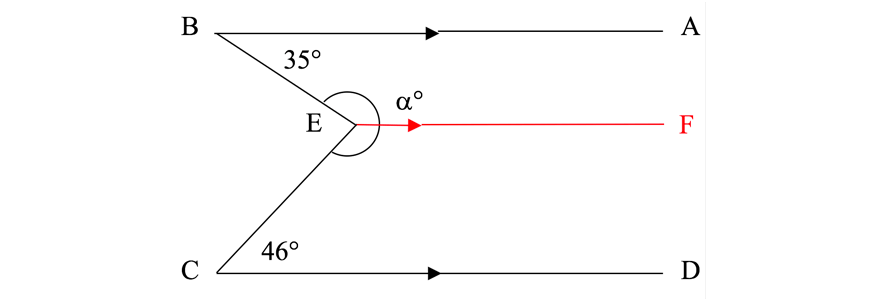\begin{align*}
\angle BEF &= 180° – 35° \ (\text{Co-interior angles on parallel lines} \ AB \ \text{and} \ EF \ \text{are supplementary}) \\
\angle BEF &= 145° \\
\angle CEF&= 180° – 46° \ (\text{Co-interior angles on parallel lines} \ CD \ \text{and} \ EF  \ \text{are supplementary}) \\
\angle CEF &= 134° \\
∴\alpha° &= \angle BEF + \angle CEF \\
\alpha°  &= 279°
\end{align*}

© Matrix Education and www.matrix.edu.au, 2023. Unauthorised use and/or duplication of this material without express and written permission from this site’s author and/or owner is strictly prohibited. Excerpts and links may be used, provided that full and clear credit is given to Matrix Education and www.matrix.edu.au with appropriate and specific direction to the original content.